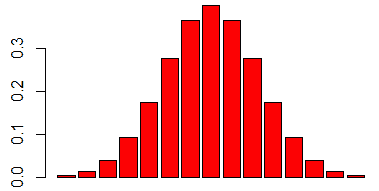# ML Wiki

## Bar Chart

Bar Chart, or Bar Plot or Bar Graph

• This is a Plot that can be useful for Exploratory Data Analysis
• It's a graphical representation of Frequency Tables
• It shows the values of your data set with bars
• height of the bar is proportional to the value it represents
• so the variables you plot must be Quantitative Variables

### In R

To create a bar chart in R

• use barplot command
r = dnorm(seq(from=-3, to=3, length=15), mean=0, sd=1)
barplot(r, col="red")## Multivariate Analysis

Bar Charts can also be used for comparing values of two and more variables

There are the following types of bar charts:

• Side-by-side bar chart
• bars are put near each other
• Stacked (Segmented) bar chart
• shows more information than other types - the total size, the proportion, etc
• Proportional stacked bar chart
• standardized version of the stacked bar chart
• makes it easier to see the Joint Distribution of variables

In R

library(openintro)
data(email)

# stacked
t = table(email$spam, email$number)
pal = c('yellow2', 'skyblue2')
barplot(t, col=pal, beside=F)

# proportional
t.prop = rbind(t[1,] / colSums(t),
t[2,] / colSums(t))
pal = c('yellow2', 'skyblue2')
barplot(t.prop, col=pal, beside=F)

# side-by-side
barplot(t, col=pal, beside=T)### Mosaic Plots

They can represent the information about the distribution better than proportional bar charts

• they use areas to represent the distribution
• e.g.